SELF 12 S.B. Karavashkin and O.N. KaravashkinaThe first salient feature of the orbital electron’s reaction to the external excitation, which the yielded solution shows us, is the absence of discrete levels of electron’s excitation. At the excitation frequency equal to that of electron’s stationary motion, the oscillation pattern is actually a circumference of large diameter, as shown in fig. 11.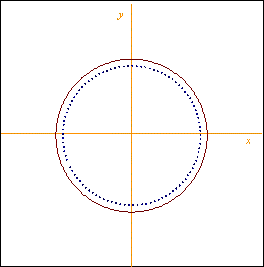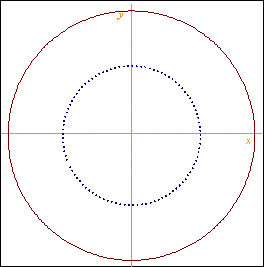a) E0 = 4,02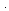10 -9 V/m                                             b) E0 = 3,2510 -8 V/m
 Fig. 11. The orbits of excited electron atE =e
 At the same time, as we can see from Fig. 11, the orbit’s radius changes with the growing strength of external field, with remaining resonance properties of atom. Because of it, when excited, the electron does not pass onto another quantified orbit, as it is conventional now; it only retains the condition of frequency resonance. And at other resonance frequencies the orbital trajectory does not remain circular, as conventional, but fully changes its configuration. This is well seen in Fig. 12 where we presented the trajectories of excited electron’s motion at the frequency of external field exceeding the natural frequency of the orbital electron.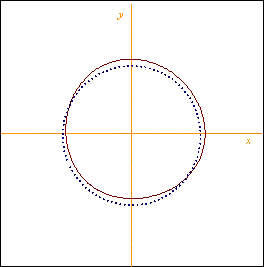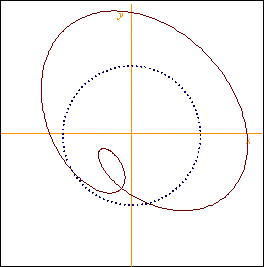a) E0 = 3,3310 -4 V/m                                            b) E0 = 2,6410 -3 V/mE = 2e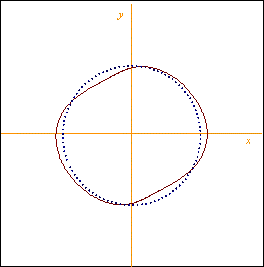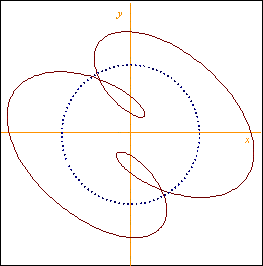a) E0 = 8,0910 -4 V/m                                             b) E0 = 6,1810 -3 V/mE = 3e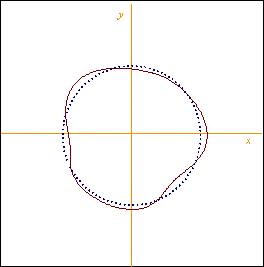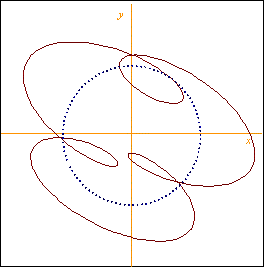a) E0 = 1,3210 -3 V/m                                            b) E0 = 1,0510 -2 V/mE = 4e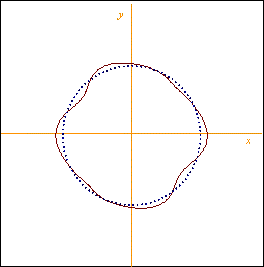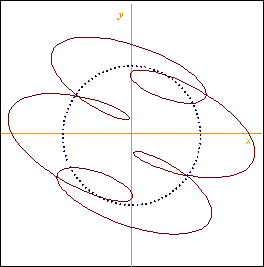a) E0 = 1,9910 -3 V/m                                            b) E0 = 1,610 -2 V/mE = 5e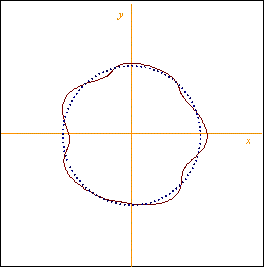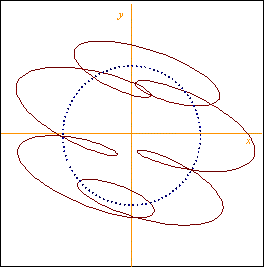a) E0 = 2,810 -3 V/m                                             b) E0 = 2,2710 -2 V/mE = 6e
 Fig. 12. Trajectories of excited electron’s motion at the frequency of exciting force exceeding the natural frequency of the orbital electron: a) in a weak field, b) in a strong field
 It is seen in the diagrams that the electron’s motion in a weak external dynamic field is the oscillations around its main orbit. With larger amplitude of the field there arise the loops of return whose quantity is by one less than the quantity of external field frequency excess over the electron’s natural frequency. These loops are formed where the electron approached the nucleus, i.e. with the radius-vector lessening. On one hand, the sequence of resonance frequencies is strongly multiple to the natural frequency of electron’s orbiting, but from the other hand, when growing multiplicity, there in the trajectory is added a full period of resonance frequency, as it is usual in wave physics. The agreement of these factors causes the sharpness of resonance peaks.

Contents: / 1 / 2 / 3 / 4 / 5 / 6 / 7 / 8 / 9 / 10 / 11 / 12 / 13 / 14 / 15 /